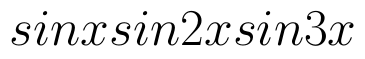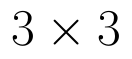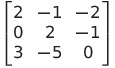Select Page

# CBSE 12 Science Answers for MCQ Maths Application of Derivatives in English

CBSE 12 Science Answers for MCQ Maths Application of Derivatives in English to enable students to get Answers in a narrative video format for the specific question.

Expert Teacher provides CBSE 12 Science Answers for MCQ Maths Application of Derivatives through Video Answers in English language. This video solution will be useful for students to understand how to write an answer in exam in order to score more marks. This teacher uses a narrative style for a question from Application of Derivatives not only to explain the proper method of answering question, but deriving right answer too.

Please find the question below and view the Answer in a narrative video format.

Question:

## Similar Questions from CBSE, 12th Science, Maths, Application of Derivatives

Question 1 : The maximum value ofis (View Answer Video)

Question 2 : The normal at the point (1, 1) on the curveis: (View Answer Video)

Question 3 : The total revenue (in Rs) received from the sale of 'x' units of a product is given by :
R(x) =3x2+36x+5.
FInd the marginal revenue when x=5, where by marginal revenue we mean the rate of change of total revenue with respect to the number of items sold at an instant. (View Answer Video)

Question 4 : Find approximate value of. (View Answer Video)

Question 5 : For all values of x, the minimum value ofis : (View Answer Video)

### Integrals

Question 1 : Evaluate the following :(View Answer Video)

Question 2 : Find :(View Answer Video)

Question 3 : Evaluate :. (View Answer Video)

Question 4 : Find :(View Answer Video)

Question 5 : Find the integral of the function. (View Answer Video)

### Determinant

Question 1 : Evaluate the determinant:. (View Answer Video)

Question 2 : Find the inverse of the matrix. (View Answer Video)

Question 3 : Evaluate the determinant:. (View Answer Video)

Question 4 : Let A be a square matrix of order, then |kA| is equal to, (View Answer Video)

Question 5 :  Evaluate the determinants:. (View Answer Video)

### Vector Algebra

Question 1 : Find the angle between the vectorsand(View Answer Video)

Question 2 : Find the area of the parallelogram whose adjacent sides are determined by the vectorsand(View Answer Video)

Question 3 : L and M are two points with position vectorsandrespectively. Write the position vectors of a point N which divides the line segment LM in the ratio 2:1 externally.   (View Answer Video)

Question 4 : Find a unit vector parallel to the sum of vectorsand(View Answer Video)

Question 5 : Find the value of x and y so that the vectorsandare equal. (View Answer Video)